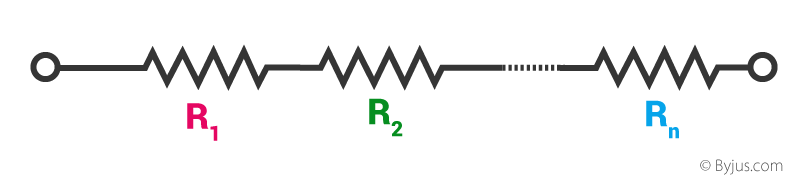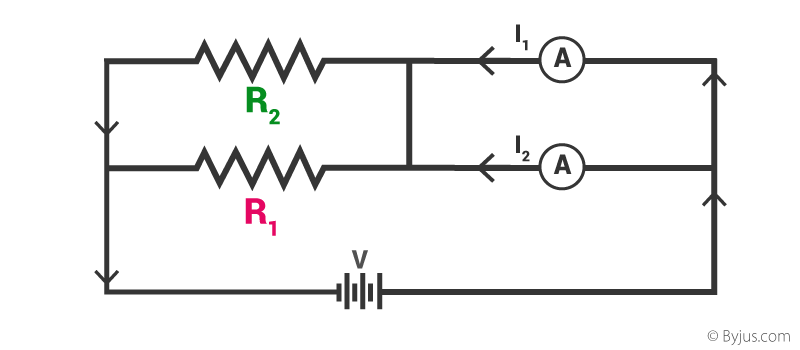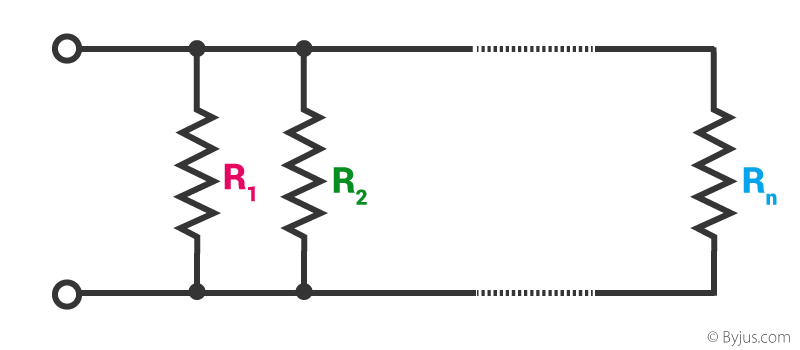# Resistors in Series and Parallel

## The Combination of Resistors – In Series and Parallel

In an electric circuit, various components can be connected either in series or in parallel manner to produce different resistive networks. Sometimes, in the same circuit, resistors can be connected in parallel and series, across different loops to produce a more complex resistive network. Resistors are said to be in series if they are joined end to end such that the same current flows through all of them. The first end of the first resistance and the last end of the last resistor is connected to the cell terminals as shown. Since the current flowing through each resistor is the same, using Ohm’s Law:

$$\begin{array}{l}V_1 = I R_1 \textup{ and } V_2 = I R_2\end{array}$$

The equivalent resistance, R must obey

$$\begin{array}{l}V = I R\end{array}$$

Since the potential difference across the two resistors must be equal to the voltage drops across each resistance,

$$\begin{array}{l}V = V_1 ~+ ~V_2\end{array}$$

$$\begin{array}{l}I R = I R_1 ~+ ~I R_2\end{array}$$

$$\begin{array}{l}R = R_1~ +~ R_2\end{array}$$Series connection across the battery### Resistors in Series

Resistors are said to be connected in series pattern, if the same amount of current flows through them. The corollary is also true, i.e., if they are connected in series, then the same amount of current flows through them.Series connection of resistors

If the resistors in a circuit are all connected in series, like the figure given above, then the total resistance of the system is given by the following relation.

$$\begin{array}{l}R_{total} = R_1~ +~R_2~ +~ …..~ +~ R_n\end{array}$$

The total resistance of the system is just the sum total of individual resistances.

For example, consider the following sample problem.

A resistor having an electrical resistance value of 100 ohms, is connected to another resistor with a resistance value of 200 ohms. The two resistances are connected in series. What is the total resistance across the system?

Here,

$$\begin{array}{l}R_1 = 100 ~Ω \textup{ and } R_2 = 200~ Ω\end{array}$$

$$\begin{array}{l}R_{total} = 100 ~+ ~200 = 300~ Ω\end{array}$$

### Resistors in Parallel

A parallel combination of two resistors is shown below:Parallel connection of resistors across voltage source

The main current I divides into two I1 and 2 which flow into the resistors R1 and R2 respectively. Both the resistors’ ends are connected with each other and hence experience the same potential difference V.

Using Ohm’s Law, we know that

$$\begin{array}{l}I_1 = \frac{V}{R_1}\end{array}$$
$$\begin{array}{l}I_2 = \frac{V}{R_2}\end{array}$$

Also, for the equivalent resistance, R:

$$\begin{array}{l}I = \frac{V}{R}\end{array}$$

Since,

$$\begin{array}{l}I = I_1~+~ I_2\end{array}$$

$$\begin{array}{l}\frac{V}{R} = \frac{V}{R_1}~ +~\frac{V}{R_2}\end{array}$$

$$\begin{array}{l}\frac{1}{R} = \frac{1}{R_1} ~+~\frac{1}{R_2}\end{array}$$

In general, any number of resistances in parallel can be written as:Parallel connection of resistors

$$\begin{array}{l}\frac{1}{R} = \frac{1}{R_1} ~+~\frac{1}{R_2}~ +~⋯~+~\frac{1}{R_n}\end{array}$$

For the problem given above, what if the resistors were connected in parallel instead of in series? What is the total resistance in that case?

$$\begin{array}{l}\frac{1}{R_{total}} = \frac{1}{100}~ + ~\frac{1}{200}\end{array}$$

$$\begin{array}{l}=\frac{(200~ +~ 100)}{20000}\end{array}$$

$$\begin{array}{l}=\frac{300}{20000}\end{array}$$

Therefore,

$$\begin{array}{l}R_{total} = \frac{20000}{300} = 66.67 ~Ω\end{array}$$

Stay tuned with BYJU’S to learn more about resistors in series, resistors in parallel and much more.

Test your knowledge on Resistor Series Parallel